# Material Procurement - Inventory levels & EOQ

CMA Poornima Madhava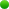on 28 March 2015

Material Procurement – Inventory Levels & EOQ

An important function of Stores is to requisition materials for replenishment. The following tools help Stores to maintain required inventory and to guide on reorder quantity: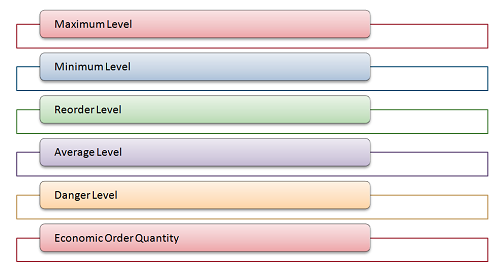Let us discuss each of the above in detail:

Maximum Level

a. It is the upper limit beyond which the quantity of any item is not normally allowed to rise

b. The objective of fixing this level is to avoid overstocking so that the working capital is not unnecessarily blocked

c. The level is fixed after taking into consideration the rate of consumption, storage space available, lead time for delivery, nature of material, working capital required, inventory carrying cost, market trends, government restrictions, economic order quantity etc

d. It is computed using the formula

MxL = RL + RQ – (MnC x MnRP)

Where,

MxL = Maximum level

RL = Reorder level

RQ = Reorder quantity

MnC = Minimum consumption

MnRP = Minimum reorder period

Minimum Level

a. It is the lower limit below which the stock of any item should not normally be allowed to fall

b. It is also known as safety/buffer stock and is fixed with the objective of avoiding shortage of materials, thus helping in protecting against stock out

c. Factors which are considered while fixing this level are average rate of consumption, lead time required for replenishment etc

d. It is calculated using the formula

MnL = RL – (AC x ARP)

Where,

MnL = Minimum level

RL = Reorder level

AC = Average consumption

ARP = Average reorder period

Reorder Level

a. It is fixed between the minimum and maximum levels

b. When the stock level reaches this point, action is required to be initiated for purchase of material

c. This level is slightly more than minimum level and helps to guard against abnormal usage, abnormal delay in supply etc

d. It is computed using the formula

RL = MxC x MxRP

Where,

MxC = Maximum consumption

MxRP = Maximum reorder period

Average Level

a. It is the average of maximum and minimum level

b. It is computed using the formula

AL = (MxL + MnL)/2 or

AL = MnL + ½ RQ

Where,

AL = Average level

MxL = Maximum level

MnL = Minimum level

RQ = Reorder quantity

Illustration 1: From the following data, calculate reorder level, minimum level, maximum level, average stock level:

 Particulars Item1 Maximum consumption per week 75 units Average consumption per week 50 units Minimum consumption per week 25 units Reorder period 4-6 weeks Reorder quantity 400 units

Solution:

 Reorder level MxC x MxRP 75 x 6 450 units Minimum level RL – (AC x ARP) 450 – (50 x 5) 200 units Maximum level RL + RQ – (MnC x MnRP) 450 + 400 – (25 x 4) 750 units Average level (MxL + MnL)/2 (750 + 200)/2 475 units

Danger Level

a. It is fixed below the minimum level and normal stock should not fall below the minimum level

b. If the stock levels reaches danger level, urgent action to be taken to replenish stock to prevent stock out

c. It is computed using the formula

DL = MnC x ERP

Where,

DL = Danger level

MnC = Minimum consumption

ERP = Emergency reorder period

Economic Order Quantity

a. It helps to ascertain the quantity to be purchased of a particular material

b. It is the most desirable quantity to be ordered at which both ordering and carrying costs will be minimum

c. Ordering cost is the cost of placing a purchase order which also includes costs like handling/transportation costs, stationery costs, costs incurred for inviting quotations/tenders etc. More the frequency of order, more is the ordering cost

d. Carrying cost is the inventory carrying cost like warehouse charges, insurance, lighting, losses due to handling, spoilage, breakage etc and also the amount of interest lost due to investment in inventory. Carrying cost increases with the increase in the quantity of the material

e. EOQ is computed using the formula

EOQ = √(2ab/cs)

Where,

a = Annual consumption in units

b = Buying cost per order

c = Cost per unit

s = Cost of carrying inventory

Illustration 2: From the following data, compute economic order quantity:

Annual consumption = 20,000 units

Buying cost per order = Rs.10

Cost per unit = Rs.100

Cost of carrying inventory = 10% of cost

Solution:

Given,

a = 20,000

b = 10

c = 100

s = 10 (10% of c)

EOQ = √(2ab/cs) = √[(2 x 20000 x 10)/10] = 200 units

Tags :

Category Accounts
Other Articles by -

Report Abuse

### Popular Articles#### GST relaxations during COVID period#### Due date of filing Return of Income#### Relief Measures declared by CBIC and CBDT in Indirect Tax and Direct Tax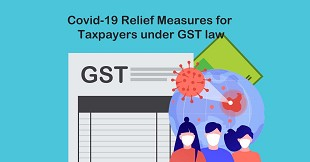#### Covid-19 Relief Measures for Taxpayers under GST law#### A Brief Explanation Of Relaxations Announced By MCA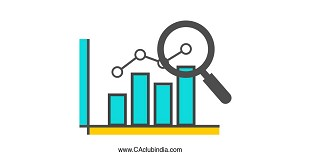#### Passout percentage of CA Final Result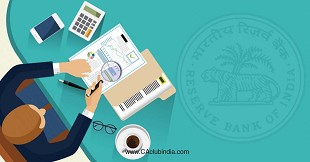#### RBI set parameters for appointing Bank Auditor for FY 2021-22 and onwards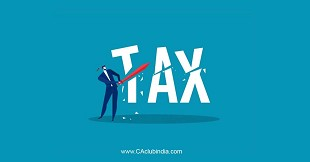#### Deductions under Chapter VIA

CCI Articles